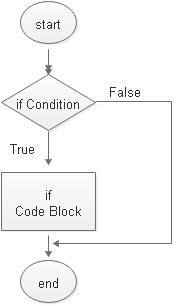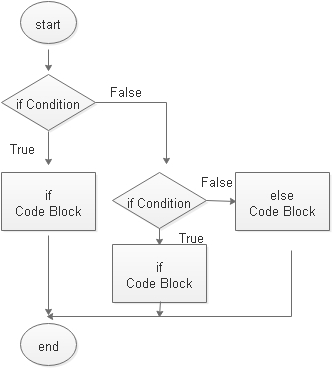# if else condition checking

Syntax of while loop``````if ( condition ){
// code block to execute
}``````
condition : Check the logic and it should return true or false.

The code block is executed only if the condition is true

## Example 1

`````` 	int mark=86;
if ( mark >80) {
System.out.println("More than 80");
}``````
Output
``More than 80``

## Understanding condition

In place of using integer variable , we will use one Boolean variable with initial value set to true.
``````   	boolean my_condition=true;

if( my_condition ) {
System.out.println(my_condition);
}``````
Output is
``true``

## if else

We will execute another code block by using else if our condition returns false.``````int mark=76;
if ( mark >80) {
System.out.println("More than 80");
}
else {
System.out.println("Less than 80");
}``````

## if else if

If condition returns false then we can add one more condition check by using else if.
``````int mark=76;
if ( mark >80) {
System.out.println("More than 80");
}
else if(mark>50) {
System.out.println("More than 50");
}``````

## if else if elseExample
``````int mark=46;
if ( mark >80) {
System.out.println("More than 80");
}
else if(mark>50) {
System.out.println("More than 50");
}
else {
System.out.println("Less than 50");
}``````
Example
``````	   	int mark=46;
if ( mark >=80) {
System.out.println("80 or more");
}
else if(mark>=50) {
System.out.println("50 or more");
}
else if(mark >=30 ){
System.out.println("30 or more");
}``````

## Nested if

Find the greatest of three given numbers.
``````	   	int i=11,j=10,k=9;
if ( j>i) {
if(j>k) {
System.out.println("Highest:" + j);
}
else {
System.out.println("Highest:" + k);
}
}
else if(i>k){
System.out.println("Highest:"+ i);
}
else {
System.out.println("Highest:" + k);
}``````

## Subscribe

* indicates required
Subscribe to plus2netplus2net.com